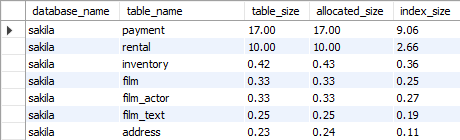## List tables by the size of data and indexes in MySQL database

Article for:

Query below returns tables in a database with space they use and space used by indexes ordered from the ones using most.

## Notes

For InnoDB storage engine, column data_length represent size of clustered index which contains all data. Results for InnoDB from index_length and data_length are only approximation.

## Query

``````select table_schema as database_name,
table_name,
round(1.0*data_length/1024/1024, 2) as data_size,
round(index_length/1024/1024, 2) as index_size,
round((data_length + index_length)/1024/1024, 2) as total_size
from information_schema.tables
where table_schema not in('information_schema', 'mysql',
'sys', 'performance_schema')
-- and table_schema = 'your database name'
order by total_size desc;
``````

## Columns

• database_name - database (schema) name
• table_name - table name
• data_size - data size in megabytes
• index_size - size of table all indexes in megabytes
• total_size - total size, data and indexes

## Rows

• One row represents one table
• Scope of rows: all tables in database with specific storage engine
• Ordered by total table size

## Sample results